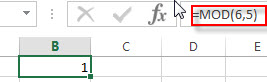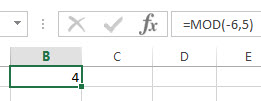# Excel MOD Function

This post will guide you how to use Excel MOD function with syntax and examples in Microsoft excel.

### Description

The Excel MOD function returns the remainder of two numbers after division. So you can use the MOD function to get the remainder after a number is divided by a divisor in Excel. And the returned result will have the same sign as divisor.

The MOD function is a build-in function in Microsoft Excel and it is categorized as a Math and Trigonometry Function.

The MOD function is available in Excel 2016, Excel 2013, Excel 2010, Excel 2007, Excel 2003, Excel XP, Excel 2000, Excel 2011 for Mac.

### Syntax

The syntax of the MOD function is as below:

`=MOD (number, divisor)`

Where the MOD function arguments are:

• number – This is a required argument.The number that you want to get the remainder.
• divisor – This is a required argument. The number that you want to divide number.

Note:

• If the divisor is 0, then the MOD function will return the #DIV/0! Error.
• The MOD function returns a result in the same sign as the divisor.

### Excel MOD Function Examples

The below examples will show you how to use Excel MOD Function to get the remainder after number is divided by divisor.

1# to get the remainder of 6/5, enter the following formula in Cell B1.

`=MOD(6,5)`2# 1# to get the remainder of -6/5, enter the following formula in Cell B1.

`=MOD(-6,5)`You will see that the sign is the same as divisor.

### Related Functions

• Excel INT Function
The Excel INT function returns the integer portion of a given number. And it will rounds a given number down to the nearest integer. The syntax of the INT function is as below:= INT (number)…

### More Excel MOD Formula Examples

• Transpose Multiple Columns into One Column
You can use the following excel formula to transpose multiple columns that contain a range of data into a single column, and you can also write an Excel VBA Macro to transpose the data of range in B1:D4 into single column F quickly…
• Delete Every Other Row
To delete every other row in Excel, you need to filter alternate rows, and then select those rows, and delete them at once. So you need to create a helper column using MOD function with ROW function…
• Highlight Multiples of Specific Value
If you want to highlight numbers that are multiples of a specific number, and you need to find those numbers firstly and then you can use the conditional formatting feature to highlight it. You need to create a formula based on the MOD function….
• Create a Five Star Rating System
How do I change the five points system to five star rating in excel. How to use the conditional formatting function to create a five star rating system in excel…..
• Sum Every Nth Row or Column
If you want to sum every nth rows in Excel, you can create an Excel Array formula based on the SUM function, the MOD function and the ROW function..….
• Generate All Possible Combinations of Two Lists
You can use a formula based on the IF function, the ROW function, the COUNTA function, The INDEX function and the MOD function to get a list of all possible combinations from those two list….